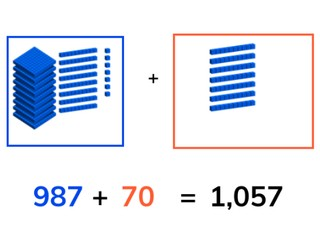Students learn to add through 1,000.8,000 schools use Gynzy92,000 teachers use Gynzy1,600,000 students use Gynzy

## General

Students learn to add through 1,000.

## Standards

CCSS.Math.Content.4.NBT.B.4

## Learning objective

Students will be able to add through 1,000.

## Introduction

Have the students first fill in the numbers to 1,000. Then you have them divide up the number 1,000 with help from the numbers provided.

## Instruction

Show the problem with a visual aid. Explain that you first count how much there is (987), then you count how much is added to it (70). Now you make the problem, 987 + 70 = . Explain that you can solve in steps by first adding up the tens values until you get to 1,007. Now you still have 50 left from the 70. Next you add this to the 1,007 to arrive at 1,057. Practice the next problem together with the students. Then you have the students solve the two problems on their own. With the problem 607 + 505, show the students how you can take jumps on the number line to add the numbers together in steps. Then show the next problem, and explain the two different ways shown in which they can solve this problem. Also ask the students if they know other ways to solve this problem in steps. The students solve the next three problems on their own. Show the problem 712 + 492. With it, show the students again how you can make jumps on the number line to add the numbers together in steps. There are also different ways to solve this problem in steps. Discuss two examples of ways to solve the problem. Then the students solve another three problems on their own. Next discuss briefly how the students can read the table to make the correct addition problem. Briefly explain how the first problem is solved and have the students solve the other problems in the table. With the story problem, show how you can determine from the story what type of problem it is. Then you get the numbers that you need from the problem and add them together. Walk through these same steps with the students for the next story problem. Of course, different strategies can be used to add the numbers together. Afterwards, the students solve two story problems on their own.

Check whether the students can add through 1,000 with the following question:
- What strategy do you use to solve a problem such as this one (692 + 521 =)?

## Quiz

Students first practice adding two numbers up to 1,000 with a visual aid. Then only the problem is shown and the students solve this. Next they must solve a story problem.

## Closing

Discuss with the students once again the relevance of the lesson. As a closing activity you can see how many problems the students can solve within 3 minutes. They can do this per row or two rows at once. You can also set more or less time on the hourglass.

## Teaching tips

When students have difficulty with adding through 1,000, they can use a number line as an aid. Explain that there are different ways in which you can take jumps on the number line. They can also use scrap paper to calculate a problem. First have them practice as much as possible with a single number to add through 1,000 (for example only the hundreds number). Then you can practice a problem with two numbers to add through 1,000.

### The online teaching platform for interactive whiteboards and displays in schools

• Save time building lessons

• Manage the classroom more efficiently

• Increase student engagement